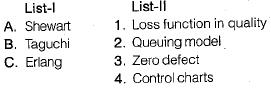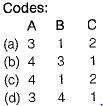Courses

# Test: Quality Analysis & Control - 2

## 10 Questions MCQ Test Topicwise Question Bank for Mechanical Engineering | Test: Quality Analysis & Control - 2

Description
This mock test of Test: Quality Analysis & Control - 2 for Mechanical Engineering helps you for every Mechanical Engineering entrance exam. This contains 10 Multiple Choice Questions for Mechanical Engineering Test: Quality Analysis & Control - 2 (mcq) to study with solutions a complete question bank. The solved questions answers in this Test: Quality Analysis & Control - 2 quiz give you a good mix of easy questions and tough questions. Mechanical Engineering students definitely take this Test: Quality Analysis & Control - 2 exercise for a better result in the exam. You can find other Test: Quality Analysis & Control - 2 extra questions, long questions & short questions for Mechanical Engineering on EduRev as well by searching above.
QUESTION: 1

Solution:
QUESTION: 2

Solution:
QUESTION: 3

### “Poka-yoke” is the Japanese term for

Solution:
QUESTION: 4

Statistical quality control considers the pattern of variation in the quality characteristics of a product due to

Solution:
QUESTION: 5

Acceptance sampling is used where
1. Inspection cost in high.
2. 100% inspection causes fatigue.
3. Inspection involves distributive testing.
Which of the above are valid?

Solution:
QUESTION: 6

Which one of the following is not the characteristic of acceptance sampling?

Solution:
QUESTION: 7

4 Which is the control chart for per cent defectives or for fraction defectives?

Solution:
QUESTION: 8

Identify the chart in which number of defects in a piece or in a sample are plotted

Solution:
QUESTION: 9

Match List-I (Scientist) with List-II (Research work) and select the correct answer using the codes given below the lists:Solution:

Taguchi-Robust design concept, orthogonal array (OA), Quality Loss function
Shewart-Control Chart
Erland - Quening model

QUESTION: 10

In a weaving operation, the parameter to be controlled is the number of defects per 10 square yards of material. Control chart appropriate for this task is

Solution: